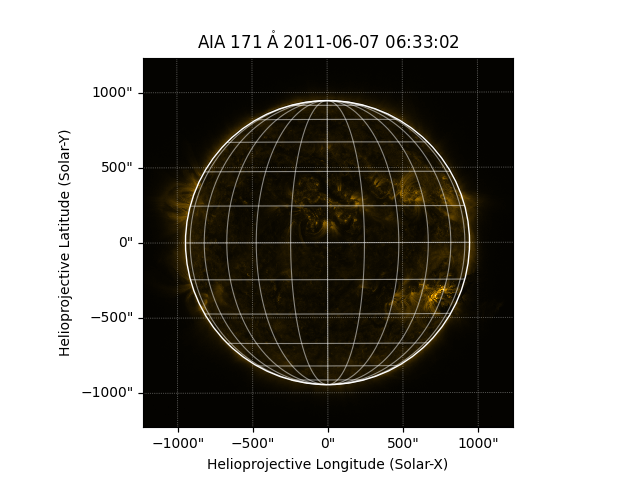# Plotting a map¶

How to create a plot of a map.

```import matplotlib.pyplot as plt

import sunpy.map
from sunpy.data.sample import AIA_171_IMAGE
```

We start with the sample data

```aiamap = sunpy.map.Map(AIA_171_IMAGE)
```

Let’s plot the result. Setting the projection is necessary to ensure that pixels can be converted accurately to coordinates values.

```plt.figure()
ax = plt.subplot(projection=aiamap)
aiamap.plot()
aiamap.draw_limb()
aiamap.draw_grid()
plt.show()
```Total running time of the script: ( 0 minutes 1.847 seconds)

Gallery generated by Sphinx-Gallery